Geometry

# Triangles - Classification by Sides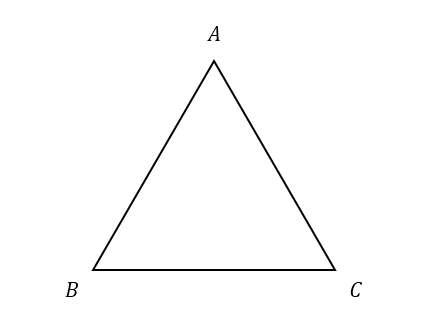If the above triangle is an equilateral triangle and the length of $\overline{AB}$ is $\lvert \overline {AB} \rvert =11 ,$ what is $\lvert \overline {AC} \rvert ?$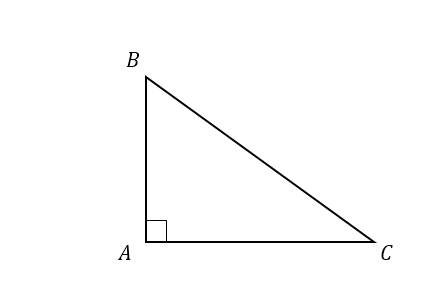If $\triangle ABC$ is a right triangle and the lengths of two sides are $\lvert \overline {AB} \rvert = 6$ and $\lvert \overline {AC} \rvert = 8 ,$ what is $\lvert \overline {BC} \rvert ?$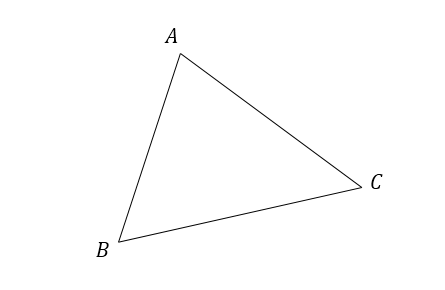$\triangle {ABC}$ is an acute triangle with side lengths $\lvert \overline {AB} \rvert = 120$ and $\lvert \overline {AC} \rvert = 160.$ If $\lvert \overline {BC} \rvert \geq \lvert \overline {AC} \rvert,$ what is the possible range of $\lvert \overline {BC}\rvert?$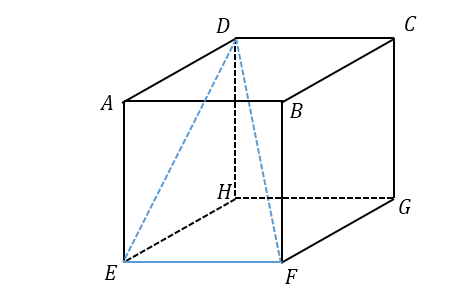Solid figure $ABCDEFGH$ is a regular cube with side length $12.$ What is the area of $\triangle DEF ?$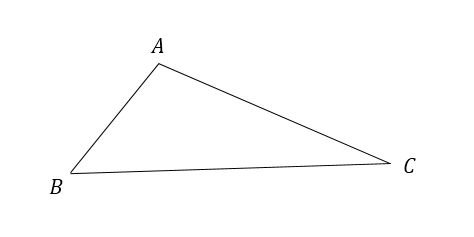Angle $\angle A$ is an obtuse angle in triangle $ABC$ with side lengths $\lvert \overline {AB} \rvert = 30$ and $\lvert \overline {AC} \rvert = 40.$ If $\lvert \overline {BC} \rvert$ is an integer and $\lvert \overline {BC} \rvert \geq \lvert \overline {AC} \rvert,$ what is the minimum value of $\lvert \overline {BC}\rvert ?$

×